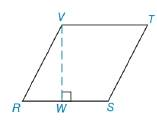Chapter 8.1, Problem 36EElementary Geometry For College St...

7th Edition
Alexander + 2 others
ISBN: 9781337614085

Solutions

Chapter
SectionElementary Geometry For College St...

7th Edition
Alexander + 2 others
ISBN: 9781337614085
Textbook Problem

In Exercises 34 to 36, provide paragraph proofs. Given: ▱ R S T V with V W ¯ ≅ V T ¯ Prove: A R S T V = ( R S ) 2To determine

To prove:

ARSTV=(RS)2 if RSTV with RW¯VT¯

Explanation

Given,

RSTV with RW¯VT¯

Let RS is the length of the base of the parallelogram RSTV.

(i.e.) b=(RS)

Where, RS¯VW¯ and RS¯||VT¯

(i.e.) RS¯=VT¯

VW¯ is the height of the parallelogram.

The area of RSTV(A)=bh unit2

=(RS¯)(VW¯)

Since,

VW¯VT¯

(i

Still sussing out bartleby?

Check out a sample textbook solution.

See a sample solution

The Solution to Your Study Problems

Bartleby provides explanations to thousands of textbook problems written by our experts, many with advanced degrees!

Get Started

Simplify: 90

Elementary Technical Mathematics

19. If and , find the following and simplify. (a) (b)

Mathematical Applications for the Management, Life, and Social Sciences

Find f'(a). f(x) = x2

Single Variable Calculus: Early Transcendentals, Volume I

In Exercises 1124, find the indicated limits, if they exist. 23. limx3x2+2x+42x23x+1

Applied Calculus for the Managerial, Life, and Social Sciences: A Brief Approach

For f(x)=exx,f(x)= a) ex b) xex1x2 c) exxexx2 d) xexxx2

Study Guide for Stewart's Single Variable Calculus: Early Transcendentals, 8th